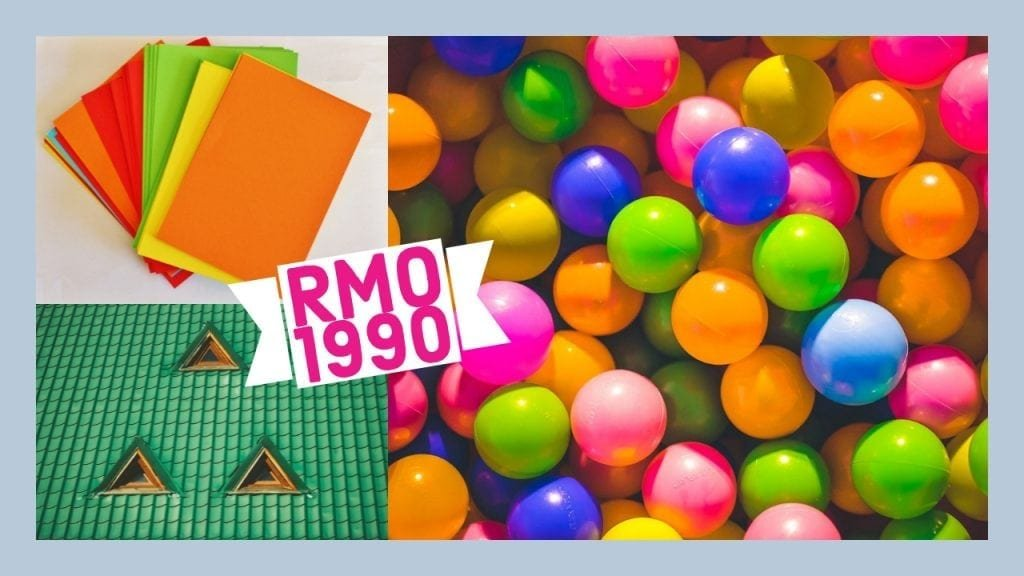How Cheenta works to ensure student success?
Explore the Back-Story

# RMO 1990 | Problems1. Two boxes contain between them 65 balls of several different sizes. Each ball is white, black, red or yellow. If you take any five balls of the same colour, at least two of them will always be of the same size (radius). Prove that there are at least three balls which lie in the same box have the same colour and have the same size (radius).
2. For all positive real numbers a, b, c prove that$\frac{a}{b+c} + \frac{b}{a+c}+ \frac{c}{a+b} \ge \frac{3}{2}$
3. A square sheet of paper ABCD is so folded that B falls on the mid-point of M of CD. Prove that the crease will divide BC in the ratio 5:3.

### Discussion

4. Find the remainder when$2^{1990}$ is divided by 1990.
5. P is any point inside a triangle ABC. The perimeter of the triangle AB +BC +CA=2s. Prove that s < AP + BP + CP < 2s.
6. N is a 50 digit number (in a decimal scale). All digits except the$26^{th}$ digit (from the left) are 1.If N is divisible by 13, find the$26^{th}$ digit.
7. A census-man on duty visited a house in which the lady inmates declined to reveal their individual ages, but said “We do not mind giving you the sum of the ages of any two ladies you may choose”. There upon the census man said, “In that case, please give me the sum of the ages of every possible pair of you”. They gave the sums as follows: 30, 33, 41, 58, 66, 69. The census-man took these figures and happily went away. How did he calculate the individual ages of the ladies from these figures?
8. If the circumcenter and centroid of a triangle coincide, prove that the triangle must be equilateral.
[/et_pb_text][/et_pb_column] [/et_pb_row] [/et_pb_section]
1. Two boxes contain between them 65 balls of several different sizes. Each ball is white, black, red or yellow. If you take any five balls of the same colour, at least two of them will always be of the same size (radius). Prove that there are at least three balls which lie in the same box have the same colour and have the same size (radius).
2. For all positive real numbers a, b, c prove that$\frac{a}{b+c} + \frac{b}{a+c}+ \frac{c}{a+b} \ge \frac{3}{2}$
3. A square sheet of paper ABCD is so folded that B falls on the mid-point of M of CD. Prove that the crease will divide BC in the ratio 5:3.

### Discussion

4. Find the remainder when$2^{1990}$ is divided by 1990.
5. P is any point inside a triangle ABC. The perimeter of the triangle AB +BC +CA=2s. Prove that s < AP + BP + CP < 2s.
6. N is a 50 digit number (in a decimal scale). All digits except the$26^{th}$ digit (from the left) are 1.If N is divisible by 13, find the$26^{th}$ digit.
7. A census-man on duty visited a house in which the lady inmates declined to reveal their individual ages, but said “We do not mind giving you the sum of the ages of any two ladies you may choose”. There upon the census man said, “In that case, please give me the sum of the ages of every possible pair of you”. They gave the sums as follows: 30, 33, 41, 58, 66, 69. The census-man took these figures and happily went away. How did he calculate the individual ages of the ladies from these figures?
8. If the circumcenter and centroid of a triangle coincide, prove that the triangle must be equilateral.
[/et_pb_text][/et_pb_column] [/et_pb_row] [/et_pb_section]

This site uses Akismet to reduce spam. Learn how your comment data is processed.

### Knowledge Partner